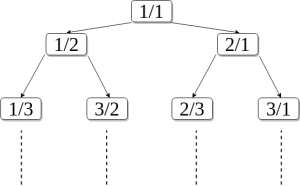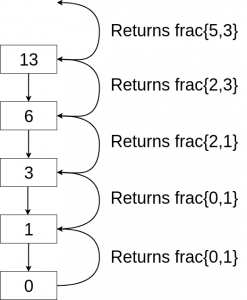# nth Rational number in Calkin-Wilf sequence

What is Calkin Wilf Sequence?
A Calkin-Wilf tree (or sequence) is a special binary tree which is obtained by starting with the fraction 1/1 and adding a/(a+b) and (a+b)/b iteratively below each fraction a/b. This tree generates every rational number. Writing out the terms in a sequence gives 1/1, 1/2, 2/1, 1/3, 3/2, 2/3, 3/1, 1/4, 4/3, 3/5, 5/2, 2/5, 5/3, 3/4, 4/1, …The sequence has the property that each denominator is the next numerator.The image above is the Calkin-Wilf Tree where all the rational numbers are listed. The children of a node a/b is calculated as a/(a+b) and (a+b)/b.

The task is to find the nth rational number in breadth first traversal of this tree.
Examples:

```Input : 13
Output : [5, 3]

Input : 5
Output : [3, 2]
```

Explanation: This tree is a Perfect Binary Search tree and we need floor(log(n)) steps to compute nth rational number. The concept is similar to searching in a binary search tree. Given n we keep dividing it by 2 until we get 0. We return fraction at each stage in the following manner:-

```    if n%2 == 0
update frac+=frac
else
update frac+=frac
```

Below is the program to find the nth number in Calkin Wilf sequence:

## C++

 `// C++ program to find the  ` `// nth number in Calkin ` `// Wilf sequence: ` `# include ` `using` `namespace` `std; ` ` `  `int` `frac[] = {0, 1}; ` ` `  `// returns 1x2 int array   ` `// which contains the nth ` `// rational number ` `int` `nthRational(``int` `n) ` `{ ` `    ``if` `(n > 0) ` `        ``nthRational(n / 2); ` ` `  `    ``// ~n&1 is equivalent to  ` `    ``// !n%2?1:0 and n&1 is  ` `    ``// equivalent to n%2 ` `    ``frac[~n & 1] += frac[n & 1]; ` `} ` ` `  `// Driver Code ` `int` `main() ` `{ ` `    ``int` `n = 13; ``// testing for n=13 ` `     `  `    ``// converting array  ` `    ``// to string format ` `    ``nthRational(n); ` `    ``cout << ``"["` `<< frac << ``","`  `         ``<< frac << ``"]"` `<< endl; ` `    ``return` `0; ` `} ` ` `  `// This code is contributed ` `// by Harshit Saini `

## Java

 `// Java program to find the nth number ` `// in Calkin Wilf sequence: ` `import` `java.util.*; ` ` `  `public` `class` `GFG { ` `    ``static` `int``[] frac = { ``0``, ``1` `}; ` ` `  `    ``public` `static` `void` `main(String args[]) ` `    ``{ ` `        ``int` `n = ``13``; ``// testing for n=13 ` ` `  `        ``// converting array to string format ` `        ``System.out.println(Arrays.toString(nthRational(n))); ` `    ``} ` ` `  `    ``// returns 1x2 int array which  ` `    ``// contains the nth rational number ` `    ``static` `int``[] nthRational(``int` `n) ` `    ``{ ` `        ``if` `(n > ``0``) ` `            ``nthRational(n / ``2``); ` ` `  `        ``// ~n&1 is equivalent to !n%2?1:0  ` `        ``// and n&1 is equivalent to n%2 ` `        ``frac[~n & ``1``] += frac[n & ``1``]; ` `         `  ` `  `        ``return` `frac; ` `    ``} ` `} `

## Python3

 `# Python program to find  ` `# the nth number in Calkin ` `# Wilf sequence: ` `frac ``=` `[``0``, ``1``] ` ` `  `# returns 1x2 int array  ` `# which contains the nth ` `# rational number ` `def` `nthRational(n): ` `    ``if` `n > ``0``: ` `        ``nthRational(``int``(n ``/` `2``)) ` `     `  `    ``# ~n&1 is equivalent to  ` `    ``# !n%2?1:0 and n&1 is  ` `    ``# equivalent to n%2 ` `    ``frac[~n & ``1``] ``+``=` `frac[n & ``1``] ` `     `  `    ``return` `frac ` ` `  `# Driver code ` `if` `__name__ ``=``=` `"__main__"``: ` `     `  `    ``n ``=` `13` `# testing for n=13 ` `     `  `    ``# converting array  ` `    ``# to string format ` `    ``print``(nthRational(n)) ` `     `  `# This code is contributed ` `# by Harshit Saini `

## C#

 `// C# program to find the nth number  ` `// in Calkin Wilf sequence:  ` `using` `System; ` ` `  `class` `GFG ` `{  ` `    ``static` `int``[] frac = { 0, 1 };  ` ` `  `    ``public` `static` `void` `Main(String []args)  ` `    ``{  ` `        ``int` `n = 13; ``// testing for n=13  ` ` `  `        ``// converting array to string format  ` `        ``Console.WriteLine(``"["` `+ String.Join(``","``,  ` `                                ``nthRational(n)) + ``"]"``);  ` `    ``}  ` ` `  `    ``// returns 1x2 int array which  ` `    ``// contains the nth rational number  ` `    ``static` `int``[] nthRational(``int` `n)  ` `    ``{  ` `        ``if` `(n > 0)  ` `            ``nthRational(n / 2);  ` ` `  `        ``// ~n&1 is equivalent to !n%2?1:0  ` `        ``// and n&1 is equivalent to n%2  ` `        ``frac[~n & 1] += frac[n & 1];  ` `         `  `        ``return` `frac;  ` `    ``}  ` `} ` ` `  `// This code is contributed by 29AjayKumar `

Output:

```[5, 3]
```

Explanation:
For n = 13,Don’t stop now and take your learning to the next level. Learn all the important concepts of Data Structures and Algorithms with the help of the most trusted course: DSA Self Paced. Become industry ready at a student-friendly price.

My Personal Notes arrow_drop_upCheck out this Author's contributed articles.

If you like GeeksforGeeks and would like to contribute, you can also write an article using contribute.geeksforgeeks.org or mail your article to contribute@geeksforgeeks.org. See your article appearing on the GeeksforGeeks main page and help other Geeks.

Please Improve this article if you find anything incorrect by clicking on the "Improve Article" button below.

Improved By : Harshit Saini, 29AjayKumar